﻿ 中部地区地方政府债务风险评估 Risk Assessment of Local Government Debt in Central China

Finance
Vol. 10  No. 06 ( 2020 ), Article ID: 38576 , 8 pages
10.12677/FIN.2020.106053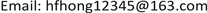Risk Assessment of Local Government Debt in Central China

Fei Huang, Weijia Wang, Qing Liu

Collage of Economics and Management, Hunan Institute of Technology, Hengyang HunanReceived: Oct. 23rd, 2020; accepted: Nov. 5th, 2020; published: Nov. 12th, 2020ABSTRACT

At present, the local government debt risk in China is generally controllable, but the situation is grim. Once it is out of control, it may evolve into a systematic risk, which will affect the national security. This paper takes six provinces in Central China as the research object, selects debt ratio and other debt risk indicators, takes the data from 2009 to 2018 as samples, and uses factor analysis method to carry out empirical research. The results show that: the debt scale of the six provinces in Central China is still expanding, and there is a certain gap in the debt risk of each province; the factors of local government debt risk are mainly divided into three categories: expenditure, income and economic benefits; Hunan has the largest debt risk due to excessive expenditure and weak financial revenue, followed by Anhui and Jiangxi.

Keywords:Local Government Debt Risk, Central Region, Risk Assessment1. 介绍

2. 文献综述

3. 模型、指标与数据

3.1. 风险评估模型

$\begin{array}{l}{x}_{1}={a}_{11}{F}_{1}+{a}_{12}{F}_{2}+\cdots +{a}_{1m}{F}_{m}+{\epsilon }_{1}\\ {x}_{\text{2}}={a}_{\text{2}1}{F}_{1}+{a}_{\text{2}2}{F}_{2}+\cdots +{a}_{\text{2}m}{F}_{m}+{\epsilon }_{\text{2}}\\ \cdots \\ {x}_{p}={a}_{p1}{F}_{1}+{a}_{p2}{F}_{2}+\cdots +{a}_{pm}{F}_{m}+{\epsilon }_{\text{p}}\end{array}$ (1)

$\begin{array}{l}{F}_{1}={\beta }_{11}{x}_{1}+{\beta }_{12}{x}_{2}+\cdots +{\beta }_{1p}{x}_{p}\\ {F}_{\text{2}}={\beta }_{\text{2}1}{x}_{1}+{\beta }_{\text{2}2}{x}_{2}+\cdots +{\beta }_{\text{2}p}{x}_{p}\\ \cdots \\ {F}_{m}={\beta }_{m1}{x}_{1}+{\beta }_{m2}{x}_{2}+\cdots +{\beta }_{mp}{x}_{p}\end{array}$ (2)

3.2. 指标与数据Table 1. Local government debt risk evaluation index system

4. 实证结果分析

4.1. 实证结果

${F}_{1}=0.205{x}_{1}+0.112{x}_{2}-0.276{x}_{3}+0.023{x}_{4}-0.079{x}_{5}+0.120{x}_{6}+0.227{x}_{7}+0.267{x}_{8}-0.129{x}_{9}-0.030{x}_{10}$

${F}_{\text{2}}=0.\text{140}{x}_{1}+0.\text{272}{x}_{2}-0.\text{023}{x}_{3}+0.\text{248}{x}_{4}+0.0\text{02}{x}_{5}-\text{0}\text{.248}{x}_{6}-\text{0}\text{.178}{x}_{7}+0.\text{001}{x}_{8}-0.\text{016}{x}_{9}+\text{0}\text{.209}{x}_{10}$

${F}_{\text{3}}=0.\text{032}{x}_{1}-\text{0}\text{.163}{x}_{2}+0.\text{037}{x}_{3}+0.023{x}_{4}-0.\text{406}{x}_{5}+0.\text{058}{x}_{6}-0.022{x}_{7}-0.120{x}_{8}+0.565{x}_{9}+0.162{x}_{10}$

$F=\text{0}\text{.405}{F}_{1}+0.382{F}_{2}+\text{0}\text{.213}{F}_{3}$Table 4. Score table of comprehensive factor of local debt risk

4.2. 债务风险根源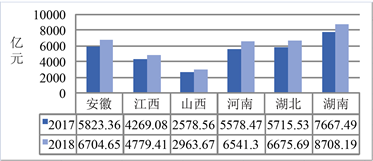(a)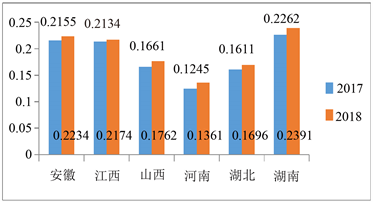(b)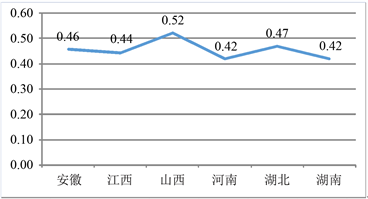(c)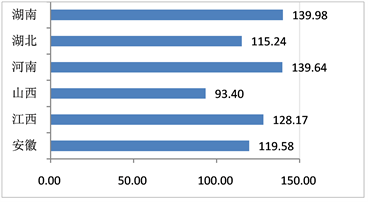(d)

Figure 1. Comparison of financial debt indicators of six provinces in Central China. (a) Debt balance; (b) Debt ratio; (c) Coefficient of financial self-sufficiency; (d) Deficit ratio

5. 结论与对策

5.1. 研究结论

5.2. 政策建议

1) 规范地方政府债务管理。根据经济发展实际需求做出相应的改变，补充和完善融资管控和债务责任追究。其次，提高对债务的重视，加强债务知情度，具备忧患意识，认识到债务和地方政府财政之间的平衡关系，不要过度举债。2) 拓宽融资渠道，推进融资平台公司改制。地方政府要鼓励建设市场化债券发行机制。3) 建立完善的风险预警机制。根据现有的债务状况通过构建风险预测模型合理预测未来几年的债务违约情况，根据预测结果，对债务结构进行优化，分散本金的偿还时间，避免地方政府短期内的集中还债情况。

Risk Assessment of Local Government Debt in Central China[J]. 金融, 2020, 10(06): 515-522. https://doi.org/10.12677/FIN.2020.106053

1. 1. 洪源, 王群群, 苏知立. 地方政府债务风险非线性先导预警系统的构建与应用研究[J]. 数量经济技术经济研究, 2018, 35(6): 95-113.

2. 2. 朱文蔚. 稳增长与防风险双重目标下的地方政府债务风险评估研究[J]. 当代经济管理, 2019, 41(2): 68-76.

3. 3. 李腊生, 耿晓媛, 郑杰. 我国地方政府债务风险评价[J]. 统计研究, 2013, 30(10): 30-39.

4. 4. 缪小林, 伏润民. 我国地方政府性债务风险生成与测度研究——基于西部某省的经验数据[J]. 财贸经济, 2012(1): 17-24.

5. 5. 李冠青. 地方政府债务风险及安全融资规模评估研究——基于山东省及其17市的实证研究[J]. 山东社会科学, 2018(10): 186-192.

6. 6. 杨子晖, 陈雨恬, 谢锐楷. 我国金融机构系统性金融风险度量与跨部门风险溢出效应研究[J]. 金融研究, 2018, 460(10): 19-37.

7. 7. 宋良荣, 侯世英. 我国地方政府性债务风险评价研究——基于资产负债视角[J]. 经济体制改革, 2018(3): 146-152.

8. 8. 洪源, 吕鑫, 张彩云. 地方政府融资平台债务绩效评价指标体系的构建[J]. 湖南大学学报(社会科学版), 2015, 29(1): 56-63.

9. 9. 洪源. 地方政府融资平台债务的可持续规模动态测算——以中部地区J市为例[J]. 中南财经政法大学学报, 2012(6): 35-42.

10. 10. 黄国桥, 徐永胜. 地方政府性债务风险的传导机制与生成机理分析[J]. 财政研究, 2011(9): 2-5.

11. 11. 靳伟凤, 张海星, 孙艺, 底萌妍. 地方政府债务风险的评价与预警机制研究——基于辽宁省的样本分析[J]. 统计与决策, 2020(19): 155-158.

12. 12. 李升, 陆琛怡. 地方政府债务风险的形成机理研究: 基于显性债务和隐性债务的异质性分析[J]. 中央财经大学学报, 2020(7): 3-16+47.

13. 13. 刘昊, 杨平宇. 地方政府债务风险识别与评估: 一个指导框架[J]. 地方财政研究, 2019(5): 21-31.

14. 14. 李温昕. 基于KMV模型的地方政府债务风险评估与管理研究[D]: [硕士学位论文]. 西安: 西北大学, 2018.

15. 15. 倪润泽. 地方政府债务风险预警体系研究[D]: [硕士学位论文]. 北京: 中国财政科学研究院, 2019.